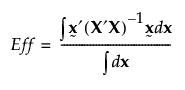Publication date: 11/29/2021

## Design Diagnostics

The Design Diagnostics outline shows D-, G-, A, and I-efficiencies for the reference design relative to the comparison designs. It also shows the Additional Run Size. Given two designs, the one with the higher relative efficiency measure is better.

Figure 16.29 Design Diagnostics for Three Designs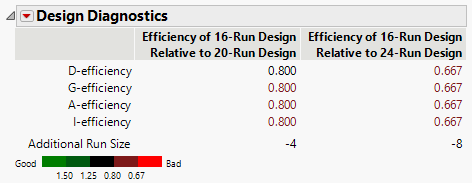Figure 16.29 shows the Design Diagnostics report for the three designs constructed in Designs of Different Run Sizes, with only main effects in the Model outline.

The values in the Design Diagnostics table are colored according to a color gradient shown under the table. You can control the color legend using the options in the Design Diagnostics red triangle menu. See Color Dashboard.

### Efficiency and Additional Run Size

Relative efficiencies for each of D-, G-, A-, and I-efficiency are shown in the Design Diagnostics report. These are obtained by computing each design’s efficiency value and then taking the appropriate ratio. The descriptions of the relative efficiency measures are given in Relative Efficiency Measures.

Additional Run Size is the number of runs in the reference design minus the number of runs in the comparison design. If your reference design has more runs than your comparison design, then the Additional Run Size tells you how many additional runs you need to achieve the efficiency of the reference design.

### Relative Efficiency Measures

#### Notation

X is the model matrix

p is the number of terms, including the intercept, in the model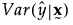is the relative prediction variance at the point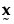. See Relative Prediction Variance.

#### Relative Efficiencies

The relative efficiency of the reference design (Ref) to the comparison design (Comp) is given by the following expressions:

D Efficiency

EffRef / EffComp, where Eff for each design is given as follows: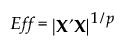G Efficiency

EffComp / EffRef, where Eff for each design is given as follows: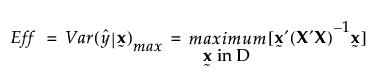Here, D denotes the design region.

Note: G-Efficiency is calculated using Monte Carlo sampling of the design space. The reported value is based on the larger of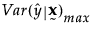or the prediction variance from the Monte Carlo sampling. Therefore, calculations for the same design might vary slightly.

A Efficiency

EffComp / EffRef, where Eff for each design is given as follows: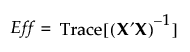I Efficiency

EffComp / EffRef, where Eff for each design is given as follows: